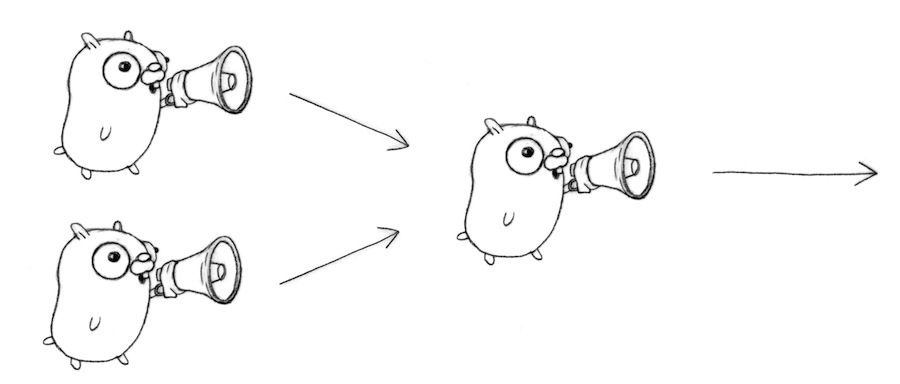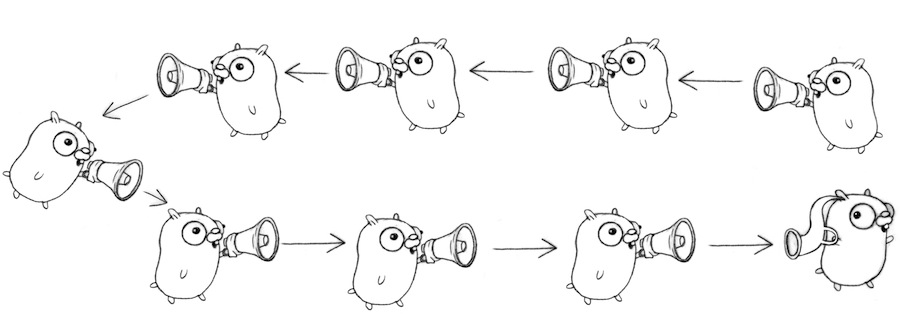# 【go语言】Goroutines 并发模式（二）

## 前言

Goroutines 并发模式（一）中，我们简单地通过boring函数的例子来粗略地阐述了通过channels来和goroutines交流的方法。在本篇中，我将从pattern的方向出发，通过对boring函数的例子进行各种改写，来讲解几种常见了goroutines的并发模式。

## 并发模式

```func boring(msg string, c chan string) {
for i := 0; ; i++ {
c <- fmt.Sprintf("%s %d", msg, i)
time.Sleep(time.Duration(rand.Intn(1e3)) * time.Millisecond)
}
}

func main() {
c := make(chan string)
go boring("boring!", c)
for i := 0; i < 5; i++ {
fmt.Printf("You say: %q\n", <-c)
}
fmt.Println("You're boring; I'm leaving.")
}```

• #### 生成器(Generator)

```func boring(msg string) <-chan string {
c := make(chan string)
go func() {
for i := 0; ; i++ {
c <- fmt.Sprintf("%s %d", msg, i)
time.Sleep(time.Duration(rand.Intn(1e3)) * time.Millisecond)
}
}()
return c
}
func main(){
c := boring("boring!")
for i := 0; i < 5; i++ {
fmt.Printf("You say: %q\n", <-c)
}
fmt.Println("You're boring; I'm leaving.")
}```

```func main() {
joe := boring("Joe")
ann := boring("Ann")
for i := 0; i < 5; i++ {
fmt.Println(<-joe)
fmt.Println(<-ann)
}
fmt.Println("You're both boring; I'm leaving.")
}```
• #### 多路复合(Multiplexing)

```func fanIn(input1, input2 <-chan string) <-chan string {
c := make(chan string)
go func() { for { c <- <-input1 } }()
go func() { for { c <- <-input2 } }()
return c
}
func main() {
c := fanIn(boring("Joe"), boring("Ann"))
for i := 0; i < 10; i++ {
fmt.Println(<-c)
}
fmt.Println("You're both boring; I'm leaving.")
}```• #### 选择(Select)

Go中的select其实和Unix/Linux下的多路复用的select在思想上有异曲同工之妙，我们可以通过Select来做很多很美妙的事情。首先，我们来改写fanin方法，把它改写为使用select的版本：

```func fanIn(input1, input2 <-chan string) <-chan string {
c := make(chan string)
go func() {
for {
select {
case s := <-input1:  c <- s
case s := <-input2:  c <- s
}
}
}()
return c
}```

• #### 超时(Timeout)

```func main() {
c := boring("Joe")
for {
select {
case s := <-c:
fmt.Println(s)
case <-time.After(1 * time.Second):
fmt.Println("You're too slow.")
return
}
}
}```

• #### 退出

```func boring(msg string, quit chan bool) <-chan string {
c := make(chan string)
go func() {
for i := 0; ; i++ {
select {
case c <- fmt.Sprintf("%s: %d", msg, i):
time.Sleep(time.Duration(rand.Intn(1e3)) * time.Millisecond)
case <-quit:
return
}
}
}()
return c
}
func main(){
quit := make(chan bool)
c := boring("Joe", quit)
for i := rand.Intn(10); i >= 0; i-- { fmt.Println(<-c) }
quit <- true
}```

• #### 菊花链(Daisy-chain)```func f(left, right chan int) {
left <- 1 + <-right
}

func main() {
const n = 100000
leftmost := make(chan int)
right := leftmost
left := leftmost
for i := 0; i < n; i++ {
right = make(chan int)
go f(left, right)
left = right
}
go func(c chan int) { c <- 1 }(right)
fmt.Println(<-leftmost)
}```0 回复

• 请尽量让自己的回复能够对别人有帮助
• 支持 Markdown 格式, **粗体**、~~删除线~~、``单行代码``
• 支持 @ 本站用户；支持表情（输入 : 提示），见 Emoji cheat sheet
• 图片支持拖拽、截图粘贴等方式上传

## 前言

Goroutines 并发模式（一）中，我们简单地通过boring函数的例子来粗略地阐述了通过channels来和goroutines交流的方法。在本篇中，我将从pattern的方向出发，通过对boring函数的例子进行各种改写，来讲解几种常见了goroutines的并发模式。

## 并发模式

```func boring(msg string, c chan string) {
for i := 0; ; i++ {
c <- fmt.Sprintf("%s %d", msg, i)
time.Sleep(time.Duration(rand.Intn(1e3)) * time.Millisecond)
}
}

func main() {
c := make(chan string)
go boring("boring!", c)
for i := 0; i < 5; i++ {
fmt.Printf("You say: %q\n", <-c)
}
fmt.Println("You're boring; I'm leaving.")
}```

• #### 生成器(Generator)

```func boring(msg string) <-chan string {
c := make(chan string)
go func() {
for i := 0; ; i++ {
c <- fmt.Sprintf("%s %d", msg, i)
time.Sleep(time.Duration(rand.Intn(1e3)) * time.Millisecond)
}
}()
return c
}
func main(){
c := boring("boring!")
for i := 0; i < 5; i++ {
fmt.Printf("You say: %q\n", <-c)
}
fmt.Println("You're boring; I'm leaving.")
}```

```func main() {
joe := boring("Joe")
ann := boring("Ann")
for i := 0; i < 5; i++ {
fmt.Println(<-joe)
fmt.Println(<-ann)
}
fmt.Println("You're both boring; I'm leaving.")
}```
• #### 多路复合(Multiplexing)

```func fanIn(input1, input2 <-chan string) <-chan string {
c := make(chan string)
go func() { for { c <- <-input1 } }()
go func() { for { c <- <-input2 } }()
return c
}
func main() {
c := fanIn(boring("Joe"), boring("Ann"))
for i := 0; i < 10; i++ {
fmt.Println(<-c)
}
fmt.Println("You're both boring; I'm leaving.")
}```• #### 选择(Select)

Go中的select其实和Unix/Linux下的多路复用的select在思想上有异曲同工之妙，我们可以通过Select来做很多很美妙的事情。首先，我们来改写fanin方法，把它改写为使用select的版本：

```func fanIn(input1, input2 <-chan string) <-chan string {
c := make(chan string)
go func() {
for {
select {
case s := <-input1:  c <- s
case s := <-input2:  c <- s
}
}
}()
return c
}```

• #### 超时(Timeout)

```func main() {
c := boring("Joe")
for {
select {
case s := <-c:
fmt.Println(s)
case <-time.After(1 * time.Second):
fmt.Println("You're too slow.")
return
}
}
}```

• #### 退出

```func boring(msg string, quit chan bool) <-chan string {
c := make(chan string)
go func() {
for i := 0; ; i++ {
select {
case c <- fmt.Sprintf("%s: %d", msg, i):
time.Sleep(time.Duration(rand.Intn(1e3)) * time.Millisecond)
case <-quit:
return
}
}
}()
return c
}
func main(){
quit := make(chan bool)
c := boring("Joe", quit)
for i := rand.Intn(10); i >= 0; i-- { fmt.Println(<-c) }
quit <- true
}```

• #### 菊花链(Daisy-chain)```func f(left, right chan int) {
left <- 1 + <-right
}

func main() {
const n = 100000
leftmost := make(chan int)
right := leftmost
left := leftmost
for i := 0; i < n; i++ {
right = make(chan int)
go f(left, right)
left = right
}
go func(c chan int) { c <- 1 }(right)
fmt.Println(<-leftmost)
}```